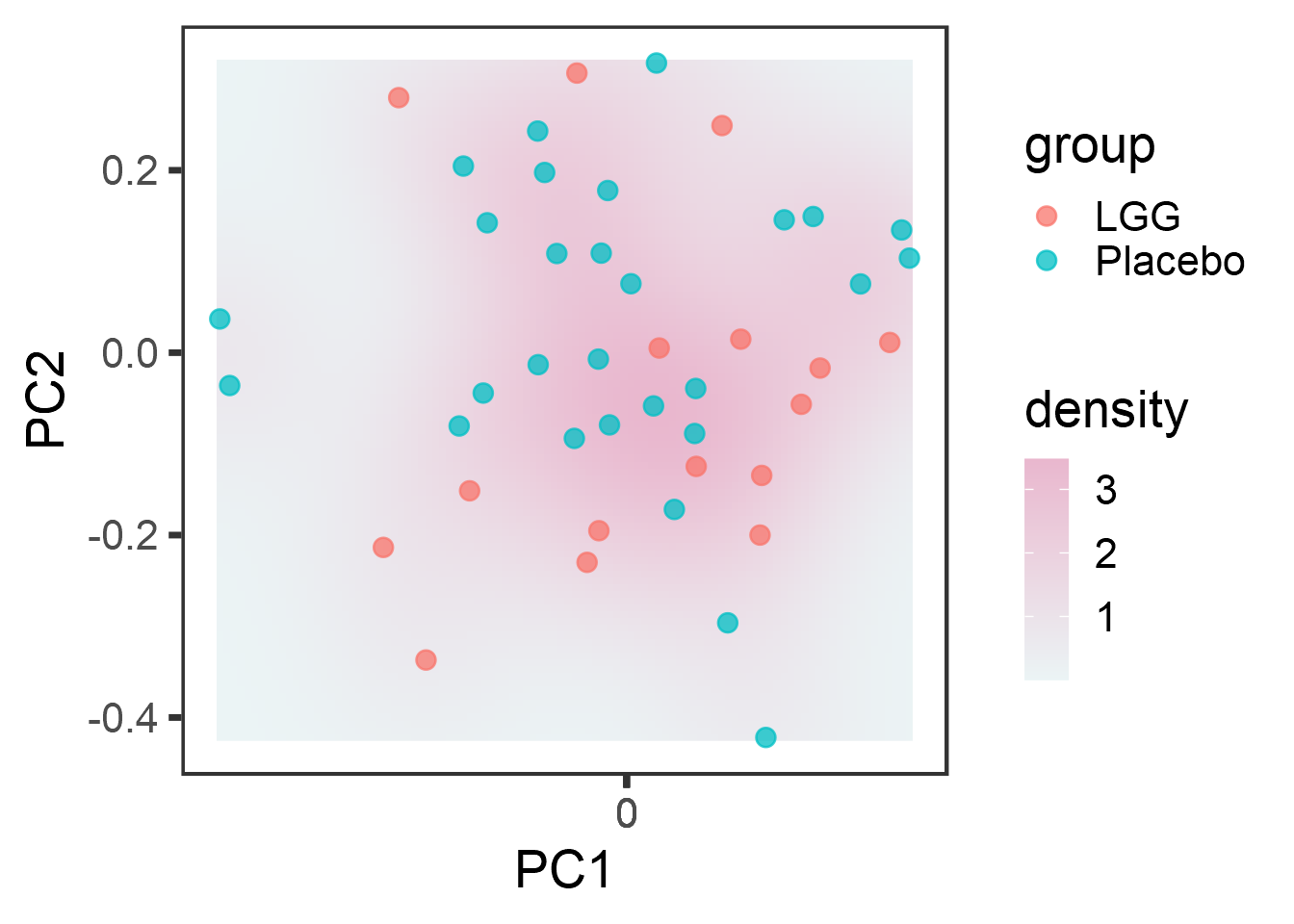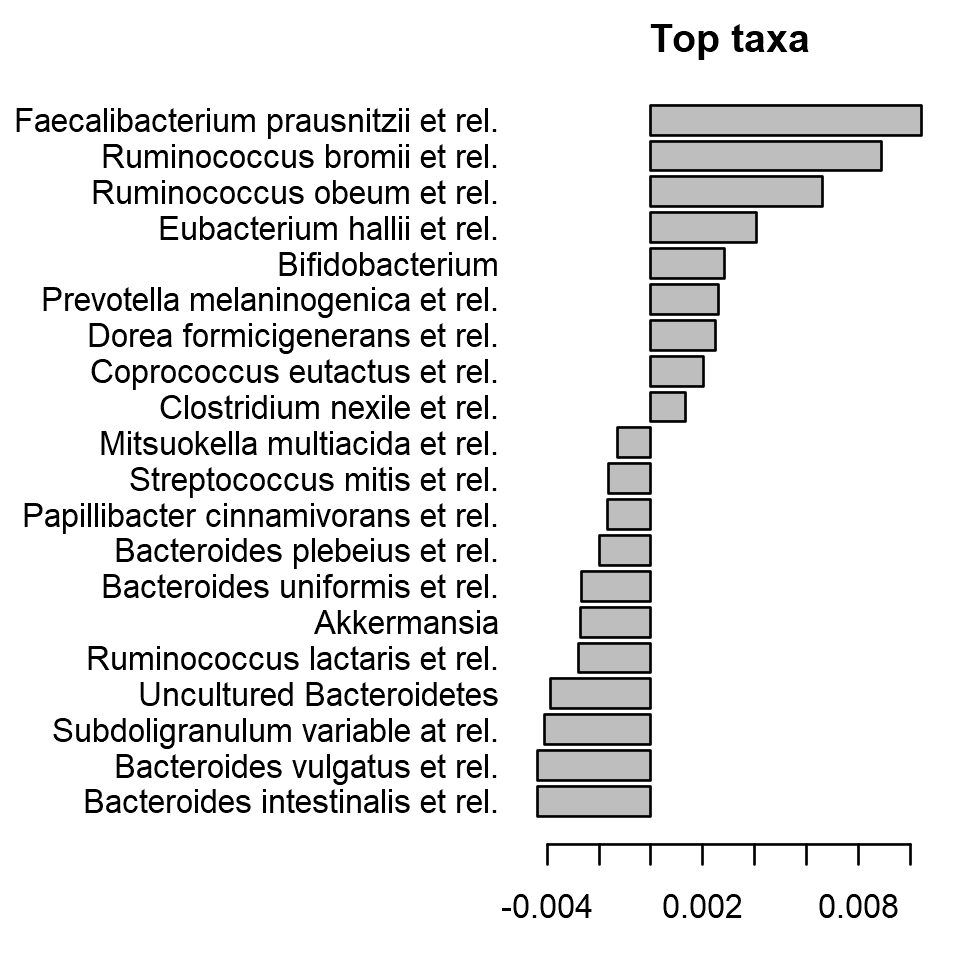## PERMANOVA for community-level multivariate comparisons

PERMANOVA quantifies multivariate community-level differences between groups.

# Load libraries
library(microbiome)
library(ggplot2)
library(dplyr)

# Probiotics intervention example data
data(peerj32) # Source: https://peerj.com/articles/32/
pseq <- peerj32$phyloseq # Rename the example data # Pick relative abundances (compositional) and sample metadata pseq.rel <- microbiome::transform(pseq, "compositional") otu <- abundances(pseq.rel) meta <- meta(pseq.rel) ## Visualize microbiome variation Visualize the population density and highlight sample groups (probiotic treatment LGG vs Placebo): p <- plot_landscape(pseq.rel, method = "NMDS", distance = "bray", col = "group", size = 3) print(p)## PERMANOVA significance test for group-level differences Now let us evaluate whether the group (probiotics vs. placebo) has a significant effect on overall gut microbiota composition. Perform PERMANOVA: # samples x species as input library(vegan) permanova <- adonis(t(otu) ~ group, data = meta, permutations=99, method = "bray") # P-value print(as.data.frame(permanova$aov.tab)["group", "Pr(>F)"])
##  0.28

## Checking the homogeneity condition

Check that variance homogeneity assumptions hold (to ensure the reliability of the results):

# Note the assumption of similar multivariate spread among the groups
# ie. analogous to variance homogeneity
# Here the groups have signif. different spreads and
# permanova result may be potentially explained by that.
dist <- vegdist(t(otu))
anova(betadisper(dist, meta\$group))
## Analysis of Variance Table
##
## Response: Distances
##           Df   Sum Sq   Mean Sq F value Pr(>F)
## Groups     1 0.000016 0.0000160  0.0043 0.9483
## Residuals 42 0.157881 0.0037591

## Investigate the top factors

Show coefficients for the top taxa separating the groups

coef <- coefficients(permanova)["group1",]
top.coef <- coef[rev(order(abs(coef)))[1:20]]
par(mar = c(3, 14, 2, 1))
barplot(sort(top.coef), horiz = T, las = 1, main = "Top taxa")